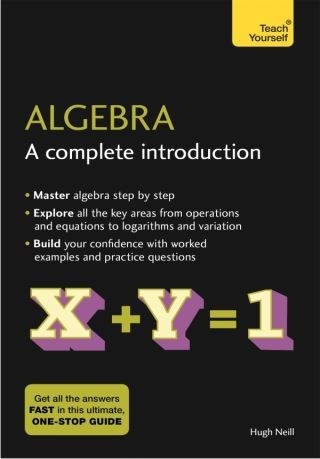## Algebra: A Complete Introduction: Teach Yourself• Description
• Author
• Info
• Reviews

### Description

Algebra: A Complete Introduction is the most comprehensive yet easy-to-use introduction to using Algebra.

Written by a leading expert, this book will help you if you are studying for an important exam or essay, or if you simply want to improve your knowledge.

The book covers all the key areas of algebra including elementary operations, linear equations, formulae, simultaneous equations, quadratic equations, logarithms, variation, laws and sequences.

Everything you will need is here in this one book. Each chapter includes not only an explanation of the knowledge and skills you need, but also worked examples and test questions.

Chapter 1: The meaning of algebra
Chapter 2: Elementary operations in algebra
Chapter 3: Brackets and operations with them
Chapter 4: Positive and negative numbers
Chapter 5: Equations and expressions
Chapter 6: Linear equations
Chapter 7: Formulae
Chapter 8: Simultaneous equations
Chapter 9: Linear inequalities
Chapter 10: Straight-line graphs; coordinates
Chapter 11: Using inequalities to define regions
Chapter 12: Multiplying algebraical expressions Chapter 13: Factors
Chapter 14: Fractions
Chapter 15: Graphs of quadratic functions
Chapter 17: Indices
Chapter 18: Logarithms
Chapter 19: Ratio and proportion
Chapter 20: Variation
Chapter 21: The determination of laws
Chapter 22: Rational and irrational numbers and surds
Chapter 23: Arithmetical and geometric sequences